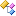﻿ Accord.Statistics.Models.Regression.Fitting Namespace# Accord.Statistics.Models.Regression.Fitting Namespace

Fitting (learning) algorithms for regression models, such as the Iterative Reweighted Least Squares for standard logistic regressors and the Lower-Bound approximator for multinomial logistic regression.Classes
ClassDescriptionIterativeReweightedLeastSquares
Iterative Reweighted Least Squares for Logistic Regression fitting.IterativeReweightedLeastSquaresTModel
Iterative Reweighted Least Squares for fitting Generalized Linear Models.LogisticGradientDescent
Stochastic Gradient Descent learning for Logistic Regression fitting.LowerBoundNewtonRaphson
Lower-Bound Newton-Raphson for Multinomial logistic regression fitting.MultinomialLogisticLearningTMethod
Gradient optimization for Multinomial logistic regression fitting.NonlinearLeastSquares
Non-linear Least Squares for NonlinearRegression optimization.NonNegativeLeastSquares
Non-negative Least Squares for MultipleLinearRegression optimization.ProportionalHazardsNewtonRaphson
Newton-Raphson learning updates for Cox's Proportional Hazards models.